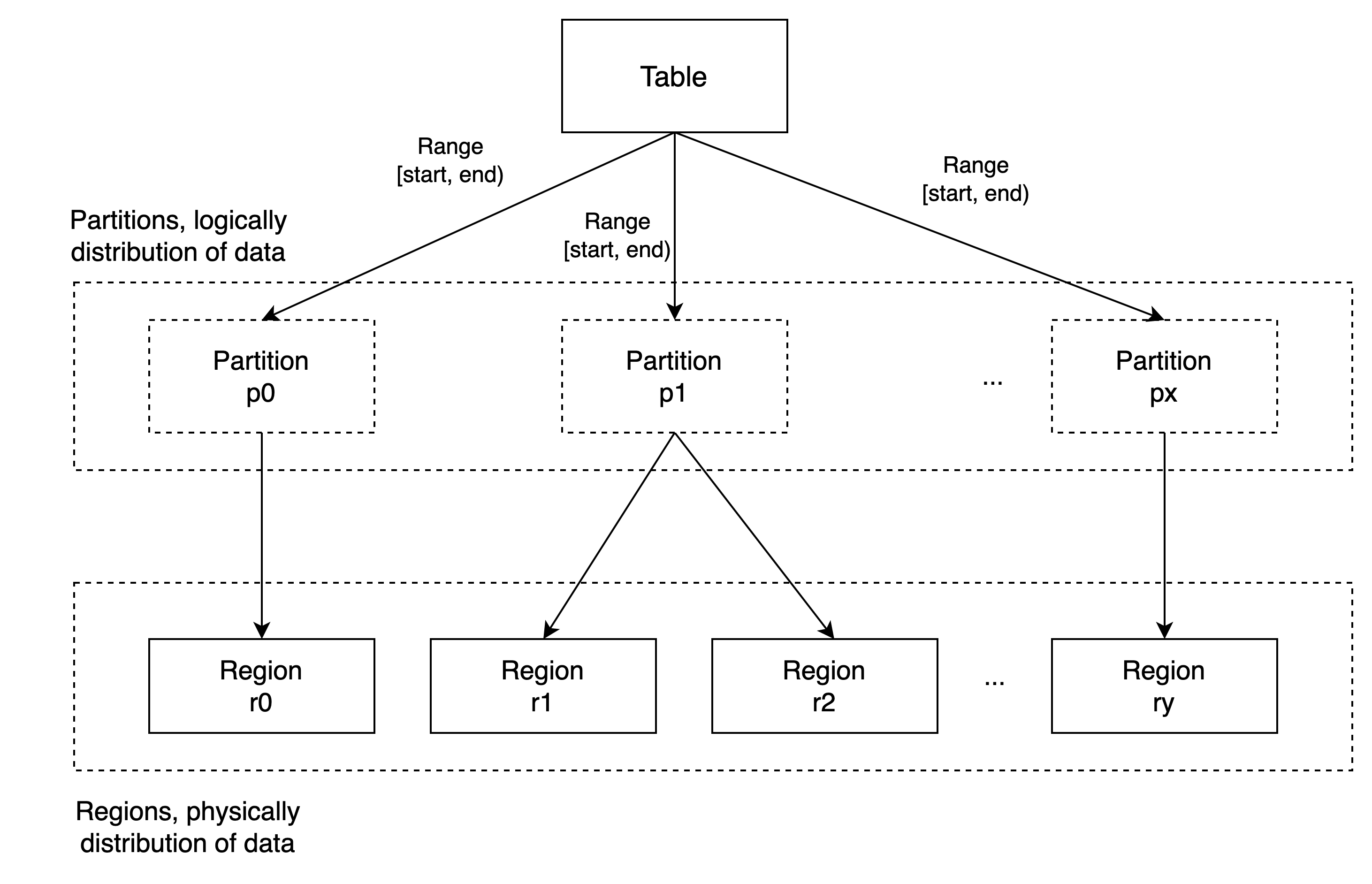# Table Sharding ​

The sharding of stored data is essential to any distributed database. This document will describe how table's data in GreptimeDB is being sharded, and distributed.

## Partition ​

In GreptimeDB, logically, data is sharded in partitions. Because GreptimeDB is using "table" to group data and SQL to query them, we borrow the word "partition", which is a concept commonly used in OLTP databases.

In GreptimeDB, a table can be horizontally partitioned in multiple ways and it uses the same partitioning types (and corresponding syntax) as in MySQL. Currently, GreptimeDB supports "RANGE COLUMNS partitioning".

In "RANGE COLUMNS partitioning", each partition includes only a portion of the data from the table, and is grouped by some column(s) value range. For example, we can partition a table in GreptimeDB like this:

sql
``````CREATE TABLE my_table (
a INT,
ts TIMESTAMP TIME INDEX,
)
PARTITION BY RANGE COLUMNS (a) (
PARTITION p0 VALUES LESS THAN (10),
PARTITION p1 VALUES LESS THAN (20),
PARTITION p2 VALUES LESS THAN (MAXVALUE),
);``````

`my_table` that we created above has 3 partitions. Partition "p0" contains a portion of data that only has rows of column "a < 10"; partition "p1" contains rows of "10 <= a < 20"; partition "p2" includes the remaining rows of "a >= 20".

Important

Value range must be strictly increased, and ends with "MAXVALUE".

Note

Currently expressions are not supported in "PARTITION BY RANGE" syntax, you can only supply column names.

## Region ​

The data within a table is logically split after creating partitions. You may ask the question " how is the data, which is physically distributed, stored in GreptimeDB? The answer is in "Region". "Region" is a group of a table's data stored together inside a Datanode instance physically. Our `metasrv` will move regions among Datanodes automatically, according to the states of Datanodes. Also, `metasrv` can split or merge regions according to their data volume or access pattern.